# Mass/Mass Stoichiometry Practice

### Problem Set 1 (from video)

C5H12 + O2 → CO2 + H2O

BALANCE THE EQUATION!

Given that the density of carbon dioxide is approximately 1.99 g/L, what volume, in liters, of carbon dioxide will be produced if 85.0 g of pentane are burned?How many molecules of water will be produced if 26.3 g of pentane are burned?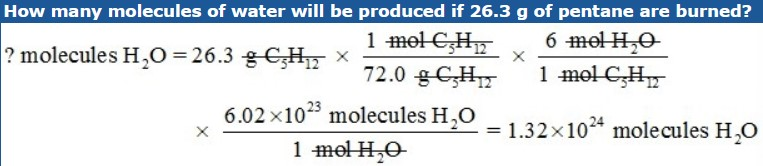### Mass/Mass Stoichiometry Problems

1. Nitrogen and hydrogen react to form ammonia gas according to the following equation. Balance the equation.

N2 + ___H2 → ___NH3

Hint

Remember the diatomic 7? The seven elements that always exist as a molecule with 2 atoms covalently bonded when found alone in nature? They are H2, N2, O2, F2, Cl2, Br2, and I2. In this reaction, both nitrogen and hydrogen will be written this way.

N2 + 3H2 → 2NH3

a. If 56.0 grams of nitrogen are used up by the reaction, how many grams of ammonia will be produced?b. How many grams of hydrogen must react if the reaction needs to produce 63.5 grams of ammonia?2. Aluminum metal reacts with zinc chloride to produce zinc metal and aluminum chloride.

Balance the equation.

__Al + ___ZnCl2 → ___Zn + ___AlCl3

2Al + 3ZnCl2 → 3Zn + 2AlCl3

a. A mass of 45.0 grams of aluminum will react with how many grams of zinc chloride?b. What mass of aluminum chloride will be produced if 22.6 grams of zinc chloride are used up in the reaction?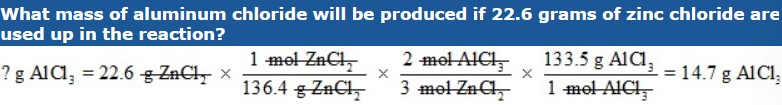3. For the reaction whose balanced equation is as follows, find the number of grams of I2 that will be formed when 300.0 g of bromine react.

2 KI + Br2 → 2 KBr + I2

Hint

Remember the diatomic 7? The seven elements that always exist as a molecule with 2 atoms covalently bonded when found alone in nature? They are H2, N2, O2, F2, Cl2, Br2, and I2. In this reaction, both bromine and iodine will be written this way.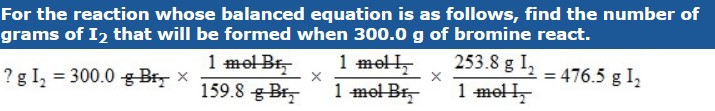4. For the reaction whose balanced equation is as follows, find the number of grams of sodium that must react to produce 42.0 grams of sodium oxide.

4 Na + O2 → 2 Na2O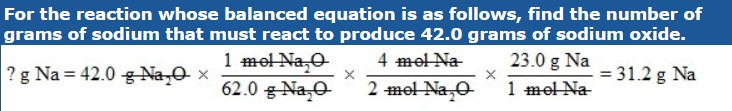5. For the reaction whose balanced equation is as follows, find how many grams of zinc phosphate will be produced by the reaction of 5.00 grams of ammonium phosphate.

3 ZnCl2 + 2 (NH4)3PO4 →Zn3(PO4)2 + 6 NH4Cl1. When mercury (II) oxide is heated, it decomposes into mercury and oxygen gas according to the following BALANCED equation.

2 HgO → 2 Hg + O2

a. Given that the density of oxygen is 1.439 g/L, how many liters of oxygen gas can be produced if 2.0 moles of mercury (II) oxide are heated?b. How many molecules of oxygen gas are produced if 25.0 g of mercury (II) oxide are heated?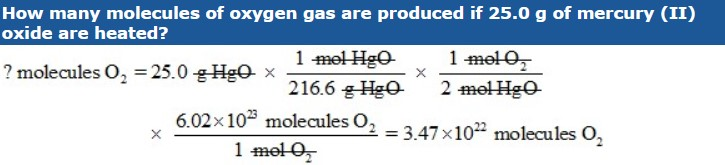2. How many molecules of sodium nitrate are produced when 20.0 g of sodium azide, NaN3, react according to the following BALANCED equation?

NaN3 + AgNO3 → AgN3 + NaNO3### Percent Yield Problems

1. Chlorobenzene, C6H5Cl, is used in the production of chemicals such as aspirin and dyes. One way that chlorobenzene is prepared is by reacting benzene, C6H6, with chlorine gas according to the following BALANCED equation.

C6H6 (l) + Cl2 (g) → C6H5Cl (s) + HCl (g)

a. What is the theoretical yield if 45.6 g of benzene react?b. If the actual yield is 63.7 g of chlorobenzene, calculate the percent yield.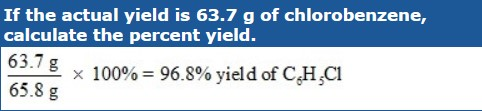2. When carbon disulfide burns in the presence of oxygen, sulfur dioxide and carbon dioxide are produced according to the following equation.

CS2 (l) + 3 O2 (g) → CO2 (g) + 2 SO2 (g)

a. What is the percent yield of sulfur dioxide if the burning of 25.0 g of carbon disulfide produces 40.5 g of sulfur dioxide?

Hint

In this problem you are given 2 quantities. First identify the quantities. You are told that you start with 25.0 g of CS2. Use this quantity to calculate the theoretical yield of SO2. You are also told that the actual yield of SO2 is 40.5. You will not use that until you have found the theoretical yield.b. What is the percent yield of carbon dioxide if 2.5 mol of oxygen react and 32.4 g of carbon dioxide are produced?

Hint

In this problem, you are given moles of oxygen. Just pick up with step 4 of the steps to solve mass-mass problems.source